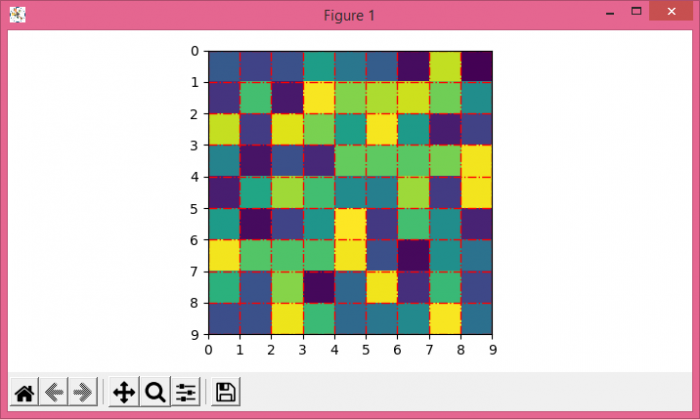# Adjusting gridlines and ticks in Matplotlib imshow

To adjust gridlines and ticks in matplotlib imshow(), we can take the following steps−

• Create data, a 2D array, using numpy.
• Using imshow() method, display data as an image.
• Set xticks and yticks using set_xticks and set_yticks method.
• To set the xticklabels and yticklabels, use set_xticklabels and set_yticklabels method.
• Lay out a grid in current line style. Supply the list of x an y positions using grid() method.
• To display the figure, use show() method.

## Example

import numpy as np
from matplotlib import pyplot as plt
plt.rcParams["figure.figsize"] = [7.00, 3.50]
plt.rcParams["figure.autolayout"] = True
data = np.random.rand(9, 9)
plt.imshow(data, interpolation="nearest")
ax = plt.gca()
ax.set_xticks(np.arange(-.5, 9, 1))
ax.set_yticks(np.arange(-.5, 9, 1))
ax.set_xticklabels(np.arange(0, 10, 1))
ax.set_yticklabels(np.arange(0, 10, 1))
ax.grid(color='red', linestyle='-.', linewidth=1)
plt.show()

## Output